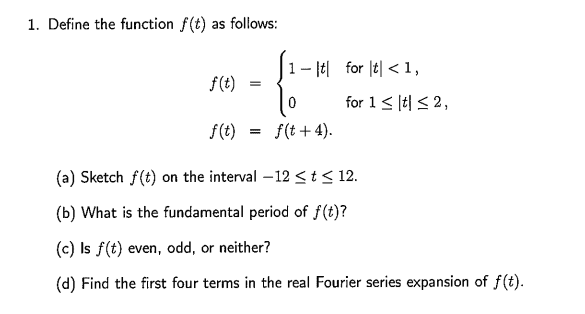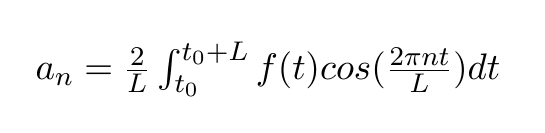# Finding Fourier Coefficient

## Homework Statement

[/B]
I am looking for help with part (d) of this question2. Homework Equations## The Attempt at a Solution

I have attempted going through the integral taking L = 4 and t0 = -2. I was able to solve for a0 but I keep having the integrate by parts on this one. I've tried it out twice and messed up. Any help would be appreciated.

BvU
Homework Helper
Hi TBD,

Well, it's nice to tell us you have attempted, but the idea is that you post your work and then we can comment, hint, etc.

LCKurtz
Homework Helper
Gold Member
You haven't told us what ##L## represents. Half period or full period? And your half range formula needs to be consistent with your choice. Also, in half range expansions, you would normally integrate from ##0## to half a period.

BvU
Homework Helper
I keep having the integrate by parts on this one
Can't be right. Show us.

Can't be right. Show us.
And you'd be right.

You haven't told us what ##L## represents. Half period or full period? And your half range formula needs to be consistent with your choice. Also, in half range expansions, you would normally integrate from ##0## to half a period.

Apologies for not being more clear. L represents the fundamental period of the function. In this case L = 4 because f(t+4) = f(t).

Sorry for not posting my workings but as it happens they were pretty much useless (I will do this in future though). I ended up splitting the integral up into 4 parts: $$\frac{1}{2} \int_{-2}^{-1}f(t) cos(\frac{\pi n t}{2}) dt + \frac{1}{2} \int_{-1}^{0}f(t) cos(\frac{\pi n t}{2}) dt + \frac{1}{2} \int_{0}^{1}f(t) cos(\frac{\pi n t}{2}) dt + \frac{1}{2} \int_{1}^{2}f(t) cos(\frac{\pi n t}{2}) dt$$
Since everything else goes to #0# we only need to consider the integral between -1 and 1. The integral from -1 to 1 is equivalent to twice the integral from 0 to 1:
$$\frac{1}{2} \int_{-1}^{1}f(t) cos(\frac{\pi n t}{2})dt = 2*\frac{1}{2} \int_{0}^{1}f(t) cos(\frac{\pi n t}{2}) dt$$and because the values of x are positive, the function ##1-|x| = 1-x## meaning we only have to integrate that and not a nasty ass modulus. In the end I got an answer of
##a_n = \frac{4}{\pi^2 n^2}##

LCKurtz
Homework Helper
Gold Member
And you'd be right.

Apologies for not being more clear. L represents the fundamental period of the function. In this case L = 4 because f(t+4) = f(t).

Sorry for not posting my workings but as it happens they were pretty much useless (I will do this in future though). I ended up splitting the integral up into 4 parts: $$\frac{1}{2} \int_{-2}^{-1}f(t) cos(\frac{\pi n t}{2}) dt + \frac{1}{2} \int_{-1}^{0}f(t) cos(\frac{\pi n t}{2}) dt + \frac{1}{2} \int_{0}^{1}f(t) cos(\frac{\pi n t}{2}) dt + \frac{1}{2} \int_{1}^{2}f(t) cos(\frac{\pi n t}{2}) dt$$
Since everything else goes to #0# we only need to consider the integral between -1 and 1. The integral from -1 to 1 is equivalent to twice the integral from 0 to 1:
$$\frac{1}{2} \int_{-1}^{1}f(t) cos(\frac{\pi n t}{2})dt = 2*\frac{1}{2} \int_{0}^{1}f(t) cos(\frac{\pi n t}{2}) dt$$and because the values of x are positive, the function ##1-|x| = 1-x## meaning we only have to integrate that and not a nasty ass modulus. In the end I got an answer of
##a_n = \frac{4}{\pi^2 n^2}##
Your last integral is correct. But you have made a mistake in your integration because it doesn't give you ##a_n = \frac{4}{\pi^2 n^2}##. All I can suggest is for you to recheck your integration since you haven't shown your steps. Also, you will need to calculate ##a_0## separately.

Last edited:
Your last integral is correct. But you have made a mistake in your integration because it doesn't give you ##a_n = \frac{4}{\pi^2 n^2}##. All I can suggest is for you to recheck your integration since you haven't shown your steps. Also, you will need to calculate ##a_0## separately.
Okay, no problem. I am right in saying ##1-|x| = 1-x## though amn't I? It's similar to an example our professor gave us which is how I made the leap. Probably just made some small error somewhere in the integration. I did calculate ##a_0## seperately and got ##\frac{1}{2}##.

LCKurtz
Homework Helper
Gold Member
Okay, no problem. I am right in saying ##1-|x| = 1-x## though amn't I?

Yes. As I said earlier, your last integral is correct with ##f(t) = 1-t##.

It's similar to an example our professor gave us which is how I made the leap. Probably just made some small error somewhere in the integration. I did calculate ##a_0## seperately and got ##\frac{1}{2}##.

You should get ##a_0 =\frac 1 4##. And your answer for ##a_n## should have ##\cos(\frac{n\pi} 2)## involved in its formula.

Last edited:
Yes. As I said earlier, your last integral is correct with ##f(t) = 1-t##.

You should get ##a_0 =\frac 1 4##. And your answer for ##a_n## should have ##\cos(\frac{n\pi} 2)## involved in its formula.

Okay must have made some massive integration errors. At least I know I have the integral right and that's half the battle :D! Thanks for your comments